# Aitken scheme

(diff) ← Older revision | Latest revision (diff) | Newer revision → (diff)
A method for computing the value at a pointof the interpolation polynomial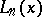with respect to the nodes, based on the successive application of the formula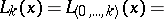(*)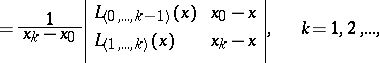where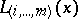is the interpolation polynomial with interpolation nodes, in particular,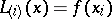(see Interpolation formula). The process of computations by means of (*) may finish if the values of two interpolation polynomials of consecutive degrees coincide in the required number of decimal places. The Aitken scheme is convenient for interpolating the values of a function given in the form of a table (of values), by renumbering the interpolation nodes in the order in which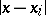increases.